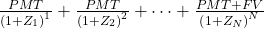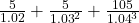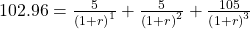Introduction to Fixed Income Valuation | IFT World
IFT Notes for Level I CFA® Program
IFT Notes for Level I CFA® Program

# Part 2

### 2.3.     Relationships between the Bond Price and Bond Characteristics

In this section, we will study the relationship between the bond price and market discount rate. The underlying principle is that a bond’s price changes whenever there is a change in market discount rate.

Inverse Effect and Convexity Effect

The bond price is inversely related to the market discount rate. As you can see from the exhibit above, when the discount rate goes down, the price of the bond increases. This is called the inverse effect. Remember that the price-yield relationship of a bond is not a straight line. Also note that price changes are not linear for the same amount of change in discount rate. At an interest rate of 20% the price of the bond is 100. If the interest rate decreases from 20% to 19% the bond price increases by 4.34%.  However, if the interest rate increases from 20% to 21%, the bond price decreases by only 4.05%. This difference in price change is called the convexity effect.

Coupon Effect

Consider three bonds (A, B, C) which have the same time to maturity but different coupon rates.

 Bond Coupon Rate Price At 20% Price At 19% % Change Price At 21% % Change A 10.00% 58.075 60.950 4.95% 55.405 –4.60% B 20.00% 100.000 104.339 4.34% 95.946 –4.05% C 30.00% 141.925 147.728 4.09% 136.487 –3.83%

Note that the bond with lowest coupon rate has the highest interest rate sensitivity. In other words, a 1% change in interest rates causes a greater % change in the price of bond A (10% coupon) relative to bonds B (20% coupon) and C (30% coupon). This is called the coupon effect.

Maturity Effect

For the same coupon rate, a longer-term bond has a greater percentage price change than a shorter-term bond when their market discount rates change by the same amount. This is called the maturity effect.

 Summary of relationship between bond prices and discount rates Inverse effect Bond price is inversely related to the discount rate. Convexity effect The percentage price change is more when discount rate goes down than when it goes up. Coupon effect For the same time-to-maturity and same change in discount rate the price of a low-coupon bond changes more than a high-coupon bond. Maturity effect For the same coupon rate and same change in discount rate the price of a long-term bond changes more than a short-term bond. Note: Low-coupon and Long-term bonds are more sensitive to changes in discount rates.

Relationship between Bond’s Price and Maturity

If the yield stays constant, bond prices change and come closer to par as time passes and as they near maturity date. This is called “pull to par”. For a premium bond, the price decreases over time to par and for a discount bond, price increases over time to par.

The exhibit below shows the constant-yield price trajectories for 5% and 10% annual coupon payment, 8-year bonds. Both bonds have a market discount rate of 7.5%. The 5% bond’s initial price is 85.3567 and the 10% bond’s initial price is 114.6433.

 Year 5% Bond 10% Bond 1 85.3567 114.6433 2 86.7585 113.2415 3 88.2654 111.7346 4 89.8853 110.1147 5 91.6267 108.3733 6 93.4987 106.5013 7 95.5111 104.4889 8 97.6744 102.3256 100.0000 100.0000

Instructor’s Note

If the yield stays constant:

• A premium bond’s price decreases to par value as its time-to-maturity approaches zero.
• A discount bond’s price increases to par value as its time-to-maturity approaches zero.
• A par bond’s value remains unchanged as it approaches maturity.

### 2.4 Pricing Bonds Using Spot Rates

Notice that we have used the same market discount rate for each of the future cash flows. Ideally, the basic approach to price a bond is to discount each cash flow at the corresponding market discount rate; hence, a sequence of discount rates must be used.

Spot rates are yields-to-maturity on zero coupon bonds maturing at the date of each cash flow. Since zero coupon bonds have no intermediate cash flows, the actual yield on a zero-coupon bond is used as the discount rate for a cash flow occurring at the same maturity date. Bond price (or value) determined using spot rates is sometimes referred to as the bond’s ‘no-arbitrage’ value. If a bond’s price differs from its no-arbitrage value, an arbitrage opportunity exists in the absence of transaction costs.

PV =where:
PMT = coupon payment
FV = par value of the bond
Z= spot rate or yield of zero-coupon bond for period 1
Z= spot rate or yield of zero-coupon bond for period 2
Z= spot rate or yield of zero-coupon bond for period N

Example

The one-year spot rate is 2%, the two-year spot rate is 3%, and the three-year spot rate is 4%. What is the price of a three-year bond that makes a 5% annual coupon payment?

Solution:

Think of the bond as a portfolio of three zero-coupon bonds with one, two and three-year maturities with yields of 2%, 3%, and 4% respectively. Draw a timeline for the cash flows.

Bond’s no-arbitrage value == 102.96

Is there a single rate (yield) for the bond that equals the present value of cash flows to its purchase price? The YTM can be calculated as:Computing for r, we get 3.93%, which is the bond’s yield-to-maturity.

Crash Courses for November CFA Level I, II exams are coming soon!
This is default text for notification bar